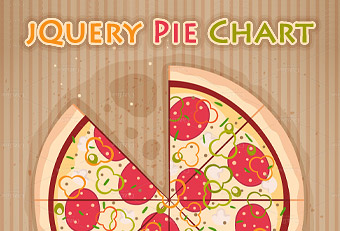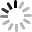# SVG 研究之路 (24) - 寫 jquery 產生圓餅圖## 原理簡介

`````` function makeSVG(tag, attrs) {
var el = document.createElementNS('http://www.w3.org/2000/svg', tag);
for (var k in attrs)
el.setAttribute(k, attrs[k]);
return el;
}
``````

`````` var i, j, path, x0, y0, x1, y1, aa, cc, bb = 0;
var all = 0;
var cx = 90;
var cy = 90;
var r = 70;
var data = [{
num: 12,
fill: '#f00'
}, {
num: 28,
fill: '#0f0'
}, {
num: 7,
fill: '#c0c'
}, {
num: 36,
fill: '#00f'
}, {
num: 7,
fill: '#c0c'
}, {
num: 17,
fill: '#0cc'
}, {
num: 12,
fill: '#cc0'
}, {
num: 7,
fill: '#c0c'
}];
``````

## 計算角度

`````` for (i = 0; i < data.length; i++) {
all = all + data[i].num;
}
for (j = 0; j < data.length; j++) {
if (j === 0) {
x0 = cx + r * Math.cos((0 * Math.PI) / 180);
y0 = cy + r * Math.sin((0 * Math.PI) / 180);
bb = bb + data.num;
cc = bb / all * 360;
x1 = cx + r * Math.cos((cc * Math.PI) / 180);
y1 = cy + r * Math.sin((cc * Math.PI) / 180);
} else if (j > 0 && j < (data.length - 1)) {
x0 = cx + r * Math.cos((cc * Math.PI) / 180);
y0 = cy + r * Math.sin((cc * Math.PI) / 180);
bb = bb + data[j].num;
cc = bb / all * 360;
x1 = cx + r * Math.cos((cc * Math.PI) / 180);
y1 = cy + r * Math.sin((cc * Math.PI) / 180);
} else {
x0 = cx + r * Math.cos((cc * Math.PI) / 180);
y0 = cy + r * Math.sin((cc * Math.PI) / 180);
x1 = cx + r * Math.cos((0 * Math.PI) / 180);
y1 = cy + r * Math.sin((0 * Math.PI) / 180);
}

if ((data[j].num / all * 360) > 180) {
aa = 'M' + cx + ' ' + cy + ',' + 'L' + x0 + ' ' + y0 + ' ' + 'A' + r + ' ' + r + ' 0 1 1 ' + x1 + ' ' + y1 + ' ' + 'Z';
} else {
aa = 'M' + cx + ' ' + cy + ',' + 'L' + x0 + ' ' + y0 + ' ' + 'A' + r + ' ' + r + ' 0 0 1 ' + x1 + ' ' + y1 + ' ' + 'Z';
}

path = makeSVG('path', {
'd': aa,
'fill': data[j].fill,
'stroke': '#fff',
'stroke-width': '2'
});
\$('#qq').append(path);

}
``````

`````` \$('path').hover(function () {
\$(this).css({
'opacity': '.5'
});
}, function () {
\$(this).css({
'opacity': '1'
});
});
``````## 小結